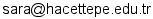# Announcements

1. Course page is on line (Feb.24)
2. Classroom HW Answer for Chapter 6 posted. (Feb.24)
3. Demonstration of Central Limit Theorem (classroom handout 1) and Demonstration of Chi-square distribution (classroom handout 2)(Feb.24)
4. Homework questions for Chapter 6 posted (Feb.24)
5. Classroom HW 2 Questions and Answers for Chapter 6 posted. (Mar.2)
6. R program HW 1 posted. Download HW Questions, Data File and Data Description. DUE March 16 (Friday) at NOON. No Late HW accepted.
7. Some notes on R Homework: 1. Data Import. Import Data as Excel causes less trouble, it seems. 2. For Task 5, write your observations with your own words. Don't just paste many graphs. PDFs (probability DENSITY function) have to be used for the observation. 3. If you are experiencing "Cannot click on Histogram" problem, try to reload data as excel. It solves the problem. (Mar.13)
9. Answers for CH6 HW questions posted.
10. Practice Questions for Chapter 7 part1 and part 2 are posted.
11. Answers for Chapter 7 part1 and part 2 practice questions are posted.
12. Practice Question and Answer for Chapter 8 part 1 is posted.
13. Midterm Exam I: April 6th Friday at 10:40. A2 and D11. Coverage: CH.6,7 and 8 (section 8.1 only). Bring a calculator which can compute sqrt, exponential, power etc. No cell phone is allowed. Necessary tables will be provided.
14. R Homework Due is postponed to April13 Friday at Noon.
15. Midterm Exam I Result is posted. (Apr.24)
16. R HW 2 Answer (Script/Output) is posted. (Apr.27)
17. HW Questions and Answers for Chapter 9 are posted. (Apr.27)
18. Midterm Exam II: May 4th Friday at 16:00. Coverage: 8.2, CH9, 10.1 and 10.2. Bring your calculator.
19. RHW3 Questions: HW/ECO240_RHW3.pdf, finalexam data (HW/finalexam.xlsx), housingdata data (HW/housingdata.xlsx ,HW/housingdata.csv) are posted. (Apr.27)
20. Practice Questions for Chapter 10 are posted. (Apr.27)
21. Practice Question for 8.2 is posted. (May 1)
22. RHW 3 due date has been extended to May 15th (Tuesday) at Noon. (May 1)
23. Answers for Sec.8.2 and CH.10 posted. (May2)
24. Practice Question  and Answers (part1 and part2) for Chapter 11 is posted.
25. Midterm Exam 2 Result posted. (May 25)

# Personnel

Instructor: Shihomi Ara-Aksoy (Email:)

# Course Information

Schedule: Fridays  9:00-11:45, D12 By appointment. The purpose of this course is to introduce important concepts of statistics, such as sampling, estimation, hypothesis testing and regression analysis. An introduction of analysis of variance (ANOVA) and time-series analysis will also be discussed. Students are expected to learn the methods of inferential statistics, the logics of hypothesis testing and regression analysis. The topics covered in this class will be important building blocks especially for the econometrics classes students will take in the third year. Make sure to understand each concept clearly.

# Texts

• Paul Newbold, William Carlson and Betty Thorne, Statistics for Business and Economics,  Seventh edition, Pearson Education, 2009.

# Lecture Schedule

## Contents

(Feb.23)

Topics:
• Introduction

### Week 2

(Mar. 2)

Topics:
• Sampling and Sampling Distribution
• Ch. 6

### Week 3

(Mar.9)

Topics:
• Confidence Interval Estimation: One Population (1)
• Ch. 7

### Week 4

(Mar.16)

Topics:
• Confidence Interval Estimation: One Population (2)
• Ch. 7

### Week 5

(Mar.23)

Topics:
• Hypothesis Test of a Single Population (1)
• Ch. 7, 9

### Week 6

(Mar.30)

Topics:
• Hypothesis Test of a Single Population (2)
• Ch. 9

### Week 7

(Apr. 6)

Topics:
• Hypothesis Test of a Single Population (3)
• Ch. 9

(Apr.13)

Midterm Exam I

### Week 9

(Apr.20)

Topics:
• Two Variable Regression Analysis (1)
• Ch.11

### Week 10

(Apr.27)

Topics:
• Two Variable Regression Analysis (2)
• Ch.11

### Week 11

(May 4)

Topics:
• Comparison of Subpopulation Means (1)
• Ch.15

### Week 12

(May 11)

Topics:
• Comparison of Subpopulation Means (2)
• Ch.15
Midterm Exam II

### Week 13

(May 18)

Topics:
• Forecasting with Time Series Models (1)
• Ch. 18

### Week 14

(May 25)

Topics:
• Forecasting with Time Series Models (2)
• Ch. 18

(TBA)

Final Exam

# Exams

• 1st Midterm exam: 8th week
• 2nd Midterm exam: 12th week
• Final exam date: to be announced